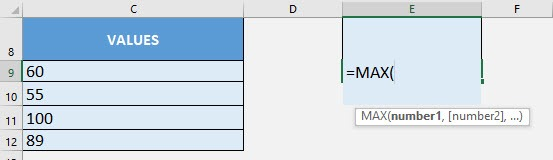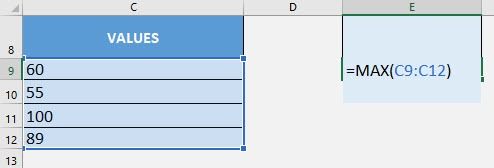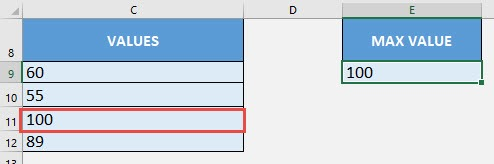What does it do?

Get the largest value from a range of values

Formula breakdown:

=MAX(number1, [number2], …)

What it means:

=MAX(a number or range of values, [additional numbers], …)

If you want to get the largest value out of a list of values, just one use of Excel’s MAX Formula gives you the answer instantly!

I explain how you can do this below:STEP 1: We need to enter the MAX function in a blank cell:

## =MAX(STEP 2: The MAX arguments:

## number1, [number2], …

Where is the list of values?

Select the cells containing the values that you want to get the maximum value from.

## =MAX(C9:C12)You now have the maximum value of 100 from the list!How to Use the MAX Formula in Excel